Courses

# Theorems of Pappus and Goldinus Mechanical Engineering Notes | EduRev

## Mechanical Engineering : Theorems of Pappus and Goldinus Mechanical Engineering Notes | EduRev

The document Theorems of Pappus and Goldinus Mechanical Engineering Notes | EduRev is a part of the Mechanical Engineering Course Engineering Mechanics - Notes, Videos, MCQs & PPTs.
All you need of Mechanical Engineering at this link: Mechanical Engineering

Pappus's Centroid Theorem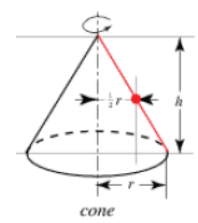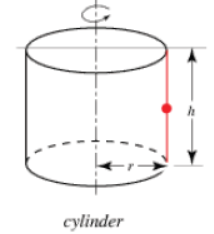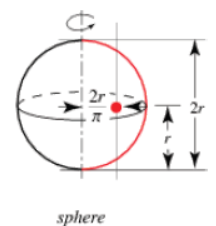The first theorem of Pappus states that the surface area Sof a surface of revolution generated by the revolution of a curve about an external axis is equal to the product of the arc length of the generating curve and the distance d1traveled by the curve's geometric centroid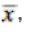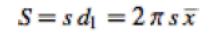(Kern and Bland 1948, pp. 110-111). The following table summarizes the surface areas calculated using Pappus's centroid theorem for various surfaces of revolution.

 solid generating curve s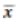S cone inclined line segment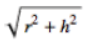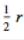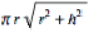cylinder parallel line segment h r 2πrh sphere semicircle πr 2π/π 4πr2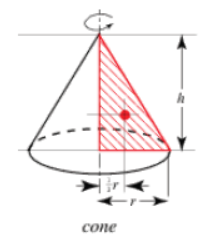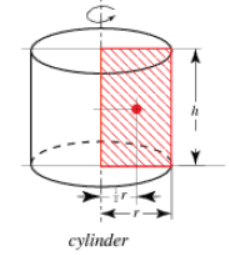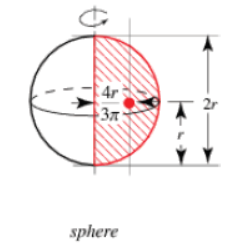Similarly, the second theorem of Pappus states that the volume V of a solid of revolution generated by the revolution of a lamina about an external axis is equal to the product of the area A of the lamina and the distance d2 traveled by the lamina's geometric centroid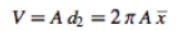(Kern and Bland 1948, pp. 110-111). The following table summarizes the surface areas and volumes calculated using Pappus's centroid theorem for various solids and surfaces of revolution.

 solid generating lamina AV cone right triangle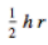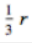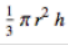cylinder rectangle hr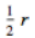πr2h sphere semicircle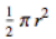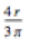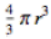Offer running on EduRev: Apply code STAYHOME200 to get INR 200 off on our premium plan EduRev Infinity!

,

,

,

,

,

,

,

,

,

,

,

,

,

,

,

,

,

,

,

,

,

;